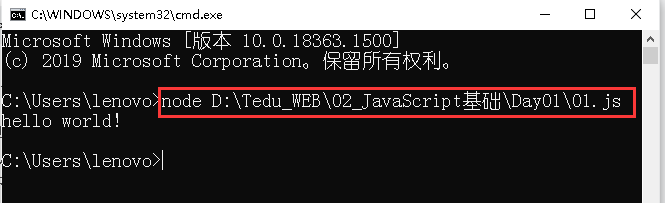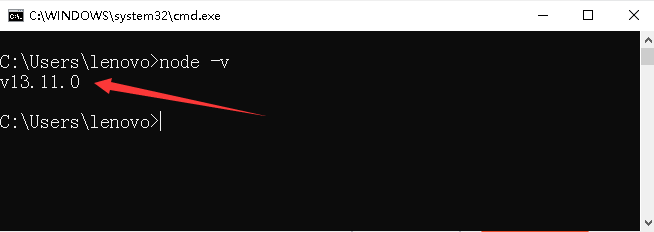# Web前端开发工程师知识体系_3_JavaScript基础（一）

2021/7/20 21:57:23 浏览：

JavaScript（简称“JS”） 是一种具有函数优先的轻量级，解释型或即时编译型的编程语言。虽然它是作为开发Web页面的脚本语言而出名，但是它也被用到了很多非浏览器环境中，JavaScript 基于原型编程、多范式的动态脚本语言，并且支持面向对象、命令式、声明式、函数式编程范式。

自ES6出世以来，JavaScript已成为世界上唯一一种可以同时包揽前后端的编程语言。

JavaScript开发环境分为浏览器端和服务器端。

所谓浏览器端就是JS代码通过浏览器自带的JS解释器来执行，典型代表就是五大浏览器：谷歌、火狐、edge、safari、欧朋。浏览器端执行需要创建一个.js文件和一个.html文件，然后将.js 文件嵌入到.html文件中。

``<script src="01.js"></script>``

服务器端则需要用到Node.js，它是运行在服务器端的开发环境。需要使用时下载安装，下载地址www.nodejs.org，执行方式也较为简单快捷，只需在命令提示符cmd下输入：node 空格  然后拖拽要运行的文件。如下：如果显示出版本号，则表示Node已成功安装。

变量是存储数据的容器；常量同变量一样用于存储数据，但常量声明以后不允许重新赋值。

声明变量使用关键字var，声明常量使用关键字const。示例：

``````//变量声明
var x = 1;//表示在内容中开辟一块空间并命名为x，把1保存至这个空间中
var y = 2;
var ename = '张三';//使用字符串时需要加引号

//常量声明
const pi = 3.14;``````

五大原始类型：数值型、字符串型、布尔型、未定义型、空

数值型number，包括二进制、八进制和十进制。

``````var n1 = 10;//10进制
var n2 = 012;//8进制
var n3 = 0xa;//16进制
var n5 = 3.1415E+2;//浮点型``````

字符串型string，被引号包含的数据即为字符串。

``````var str1 = 'abc';
var str2 = '8';``````

布尔型Boolean，只有两个值：true、false，通常用于保存只有两个状态的数据，例如是否在线、是否登录、一些运算符的结果等。

未定义型undefined，只有一个值undefined，代表一个空值，例如声明了变量未赋值。

空null，只有一个值null，类型为object，通常结合对象一起使用

``````var p = null;//定义一个变量为空
console.log(p,typeof p);//打印并用typeof检测数据类型，显示为null``````

（1）数字+字符串   数字转换为字符串后拼接
（2）数字+布尔型   布尔型转换为数字，true--1，false--0
（3）布尔型+字符串   布尔型转换为字符串后拼接
（4）undefined+数字  undefined转为NaN
（5）减乘除时，数据自动转为数值

``````var n1 = 2 + '3';//23
var n2 = 2 + true;//3
var n3 = 'zxc' + false;//zxcfalse
var n2 = '3' - 1;//2
var n3 = '2' * true;//2``````

（1）强制转换为数值型 Number( )

``````var n1 = Number("1"); //1
var n1 = Number(true); //1
var n2 = Number(false); //0
var n3 = Number(undefined); //NaN
var n4 = Number(null); //0
var n5 = Number("1a"); //NaN``````

（2）强制转换为整型 parseInt( ) ，强制将字符串和小数转为整型

``````var p1 = parseInt("3.14"); //3
var p2 = parseInt("6.18a"); //6
var p3 = parseInt("a6.18"); //NaN
var p4 = parseInt(5.9); //5``````

（3）强制转换为浮点型 parseFloat( ) ，强制将字符串转换为浮点型

``````var f1 = parseFloat("3.14"); //3.14
var f2 = parseFloat("6.18a"); //6.18
var f3 = parseFloat("6a"); //6
var f4 = parseFloat("a6.18"); //NaN``````

（4）数值和布尔型强制转为字符串 toString( )

``````var num = 5;
var str = num.toString( ); //"5"``````

在学习运算符之前，先来了解以下“表达式”的定义：由数据本身或者由运算符连接的操作数据组成的形式称为表达式，也就是说，运算符所连接的数据均称为表达式。

（1）算数运算符。即我们平常所用的加(+)、减(-)、乘(*)、除(/)，还有取余(%)、自增(++)、自减(--)。加减乘除以及取余较为简单，不做探讨。通过一组代码来理解自增和自减：

``````var a2 = 5;
var a3 = a2++;//先将a2的值赋给a3，再自增
console.log(a2,a3);//6 5``````
``````var a4 = 7;
var a5 = ++a4;//a4先自增，再赋值给a5
console.log(a4,a5);//8 8``````

可以看出，如果自增用在变量后面，则是先使用变量的值参与计算，随后再进行变量加1；而如果自增用在变量前面，则是先将变量进行自增以后再参与计算，自减同理。

（2）比较运算符

· 等于“==”只比较两者的值是否相同，全等于“===”同时比较类型和值
· 不等于“!=”只比较值是否不同，不全等于“!==”同时比较类型和值，有一个不等即为true
· 数字与字符串比较时，字符串转为数值
· 字符串之间比较时，比较首个字符的Unicode码
· NaN和任何值比较均返回false，NaN == NaN也为false

``````console.log(2 == '2');//== 只比较值的大小，都为2，所以显示true
console.log(2 === '2');//=== 比较值和类型，值虽然都为2，但前者为数值型，后者为字符串，所以显示false``````

（3）逻辑运算符

&&逻辑与，关联的两个条件都为true，结果为true

||逻辑或，关联的两个条件有一个为true结果即为true  ，

！逻辑非，取反 eg：!true = false

逻辑运算符中比较重要的为短路逻辑：

``````var a = 2;
a > 3 && console.log(num);``````

如上代码，a的值为2，逻辑与判断中，第一个表达式为a>3，而2不大于3，说明此处为false，由于这里使用的运算符为逻辑与&&，只有前后两个条件都为true时整体才为true，所以第二个表达式就没有必要再运行了，此时就是发生了短路逻辑。换句话说，短路逻辑的看重点就是在于是否执行第二个表达式。

（4）位运算符

模拟计算机底层的运算，先将数据转为2进制进行运算，运算结束后再将结果转回10进制
· & 按位与，上下两位都是1，结果为1，否则为0
·  | 按位或，上下两位有一个1则结果为1
· ^ 按位异或，上下两位不同为1，相同为0
· >> 按位右移，删除末尾的位数（原基础上除以2再取整）
· << 按位左移，在末尾补0（原基础上乘以2）

``````//按位与
console.log(3 & 5);//011 & 101 == 001 == 1
console.log(5 & 8);//0101 & 1000 == 0000 == 0
//按位或
console.log(4 | 7);//100 | 111 == 111 == 7
//按位异或
console.log(6 ^ 9);//0110 ^ 1001 == 1111 == 15
//按位右移
console.log(9 >> 1);//1001 >> 1 == 0100 == 4
//按位左移
console.log(5 << 1);//101 << 1 == 1010 == 10``````

（5）赋值运算符

所谓赋值运算，就是先进行运算，再进行赋值

``````var a = 1;
a += 3;//4
a = a + 3;//4``````

（6）三目运算符

· 一目运算符：由一个运算符连接的一个操作数据或者表达式  !  ++  --
· 二目运算符：由一个运算符连接的两个操作数据或者表达式
· 三目运算符：由两个运算符连接的三个操作数据或者表达式
格式：条件表达式 ? 表达式1 : 表达式2
如果条件表达式为true，执行表达式1
如果条件表达式为false，执行表达式2

``````var a = 1,b = 2;
a > b ? console.log('对') : console.log('错');``````

在以上函数中，a为1，b为2，首先判断a是否大于b，如果大于，则执行console.log('对')，如果小于，则执行console.log('错')。所以此语句输出结果为：错。